Lecture 22: Queues

Overview

1. The Queue Abstract Data Type
3. The Josephus Problem

Previously

Data Stuctures

• Arrays

Abstract Data Types

• Collection
• add, remove, test for containment
• Stack
• push, pop, peek

Stack Ordering

Stacks are Last In, First Out (LIFO):

• the last item pushed is next item popped

Often items should be processed in the order they arrive:

• First In, First Out (FIFO)

Examples:

• Standing in line
• first come, first serve
• Communication buffers

The Queue

Another abstract data type: the queue

Supports two basic operations:

• void enq(T item) enqueue (add) an item to the end of the queue
• T deq() dequeue (remove) item from the front queue and return it

The first item enqueued is the first to be dequeued, etc

Visualizing Enqueue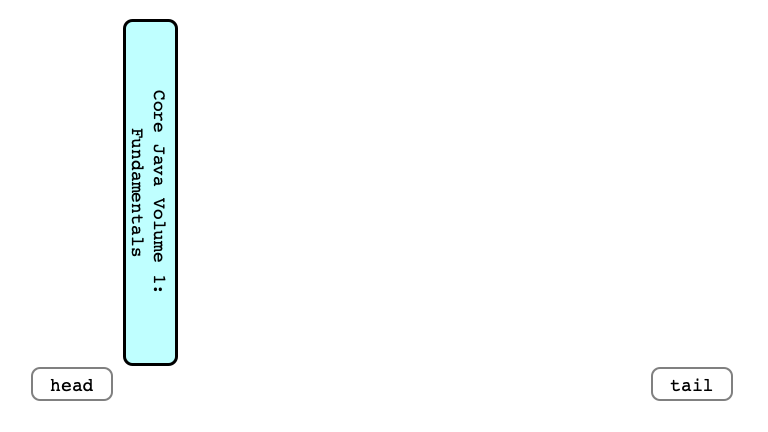Enqueue Another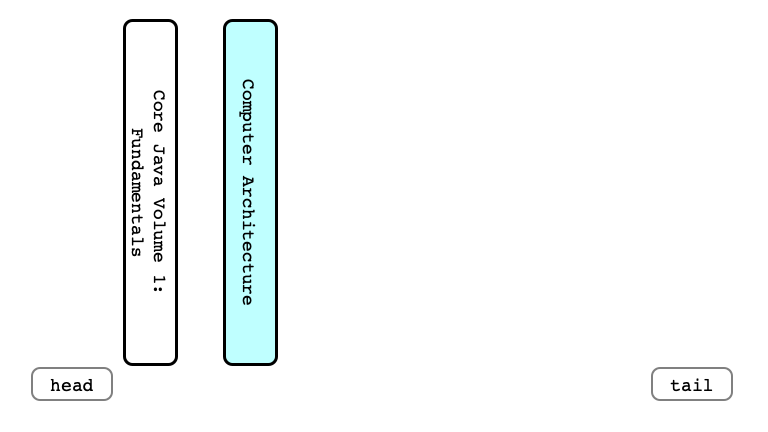And Another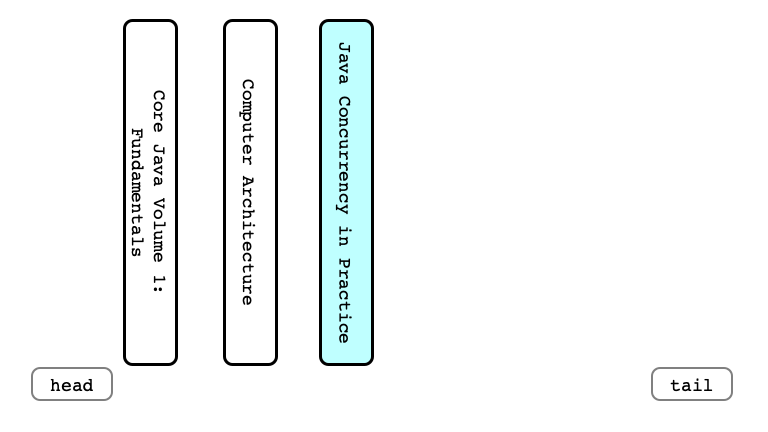Dequeue Once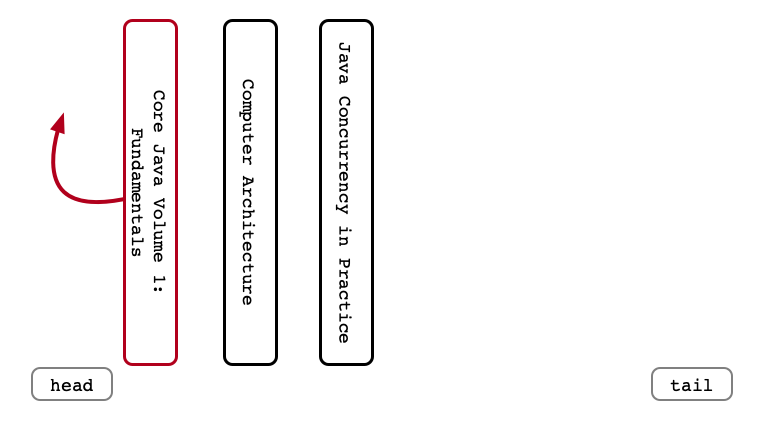Enqueue Again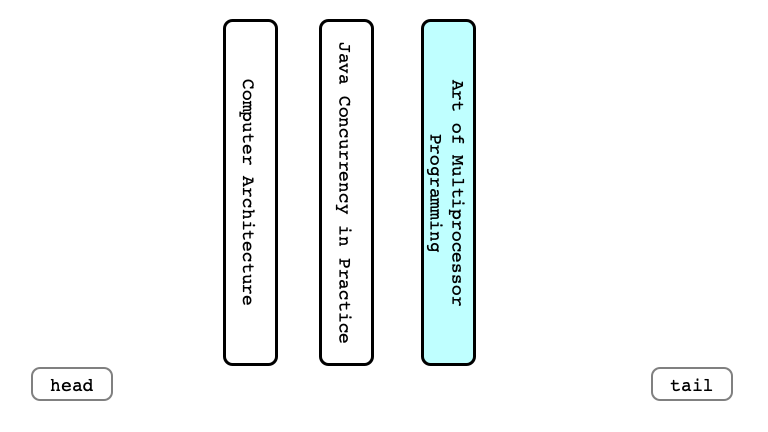Dequeue Again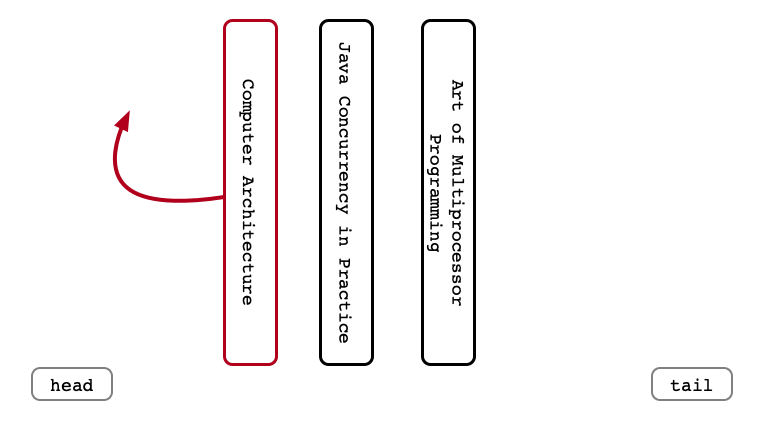Enqueue Last Time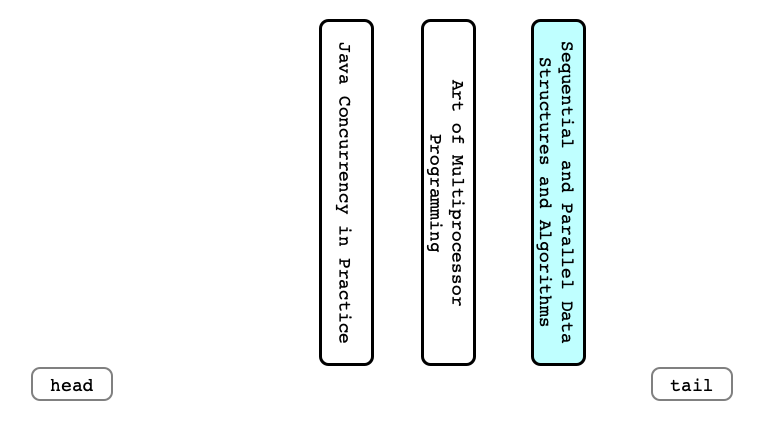Implementing a Queue

How could we implement a queue with a linked list?

A QueueListEnqueue Step 1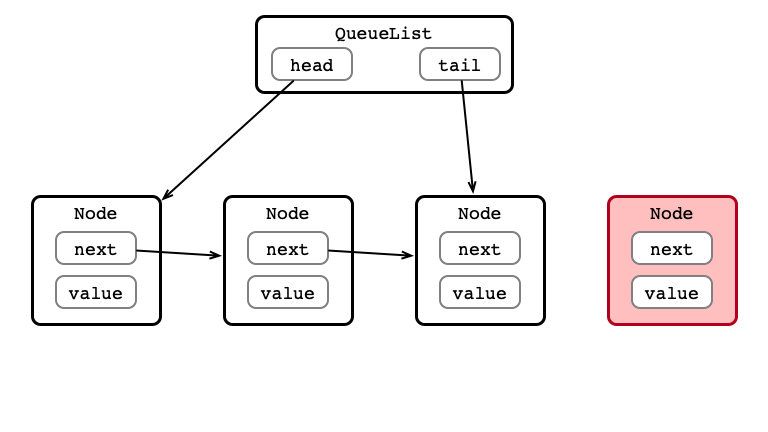Enqueue Step 2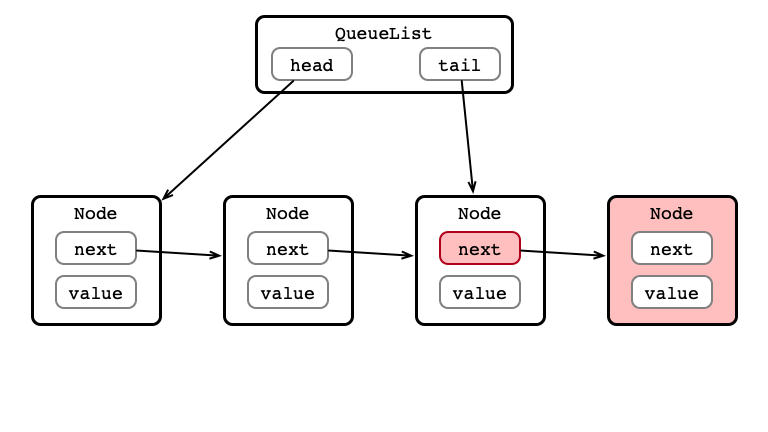Enqueue Step 3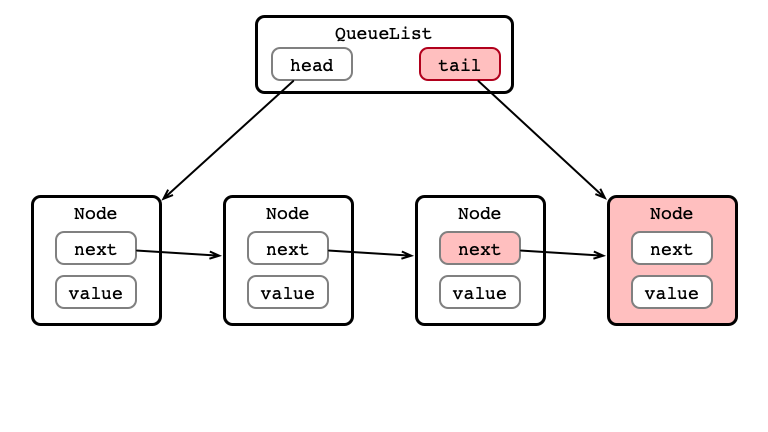Item Enqueued! Dequeue?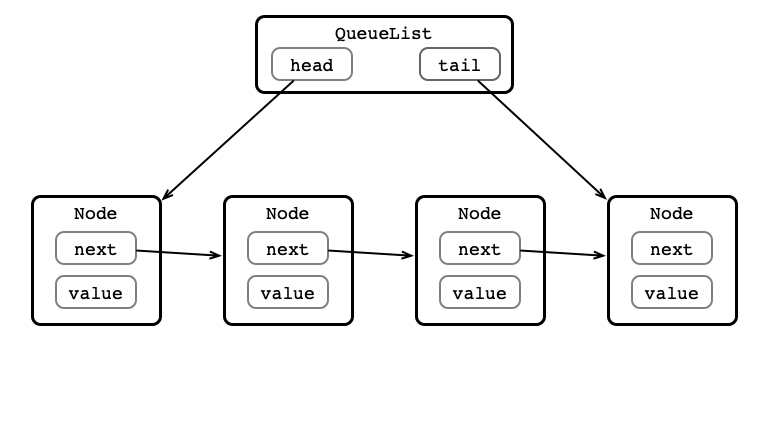Dequeue Step 1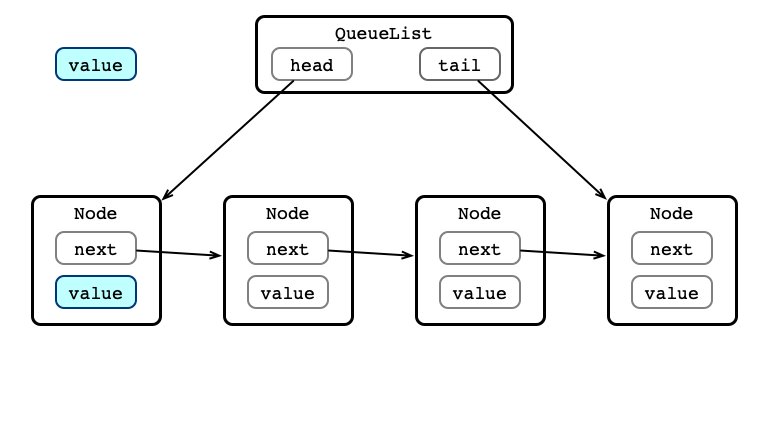Dequeue Step 2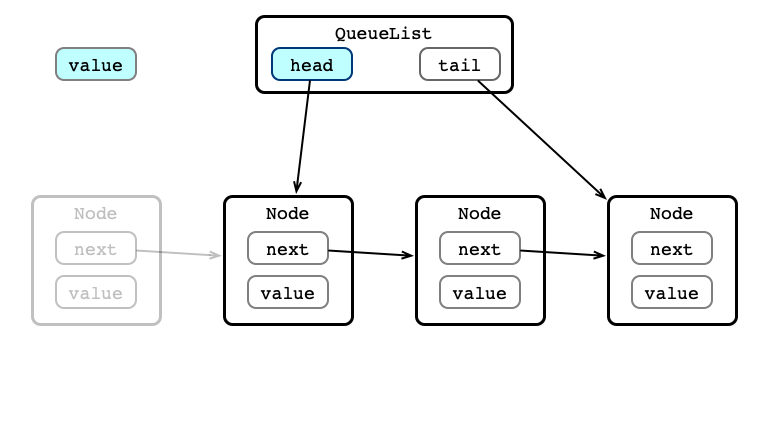Dequeue Step 3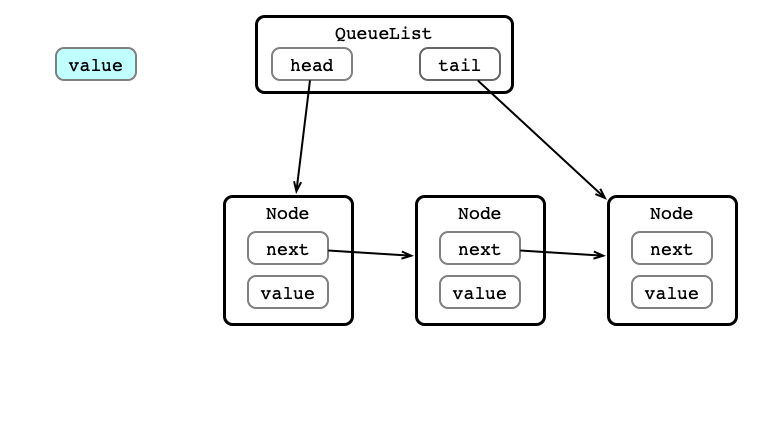A Queue Interface

public interface SimpleQueue<T> {

// Enqueue item to the queue
void enq(T item);

//Dequeue (i.e., remove and return) the first item in the queue. Returns null if the queue is currently empty
T deq();

// Determine if the queue is currently empty
boolean isEmpty();
}

A Queue Implementation

public class QueueList<T> implements SimpleQueue<T>, Iterable<T> {
private Node tail = null;

@Override
public void enq(T item) {
Node nd = new Node(item);

tail = nd;
return;
}

tail.next = nd;
tail = nd;
}

@Override
public T deq() {
return null;
}

return item;
}

@Override
public boolean isEmpty() {
}

public T peek() {
return null;
}

}

class Node {
private Node next;
private T item;

public Node(T item) {
this.item = item;
}
}

public Iterator<T> iterator() {
return new ListIterator();
}

class ListIterator implements Iterator<T> {
Node curr;

public ListIterator() {
}

public boolean hasNext() {
return (curr != null);
}

public T next() {
if (curr == null) {
return null;
}

T item = curr.item;
curr = curr.next;
return item;
}
}

}

The Josephus Problem

• Historical problem inspired by Josephus’ The Jewish War
• We will consider a less gruesome retelling of the problem

Josephus’ Manicure Problem

• $n$ people determine to give each other manicures
• they only have 1 set of tools/supplies
• only one person can give another a manicure at a time
• once a person receives a manicure, they leave

Setup:

• people seated at a round table
• labeled sequentially clock-wise from $1$ to $n$

Illustration $n = 5$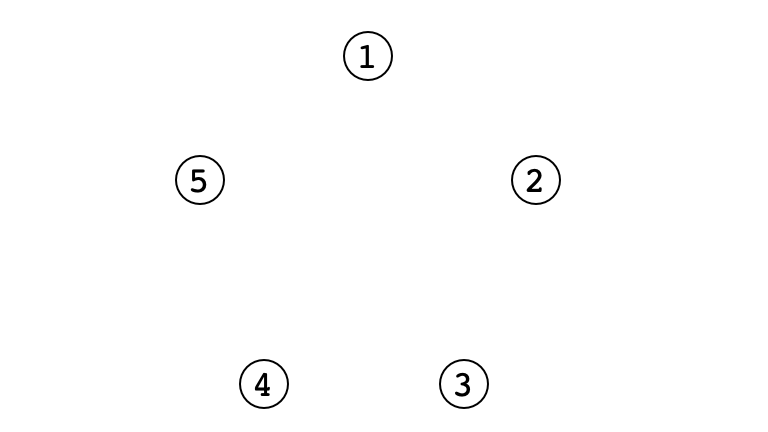A System

• the current manicurist gives a manicure to the person seated to their left
• after the manicure, person to their left leaves
• the manicurist hands the tools to the next person to their left who becomes the next manicurist
• repeat until all but one person receives a manicure

Initially, person 1 is manicurist.

Example $n = 5$Example $n = 8$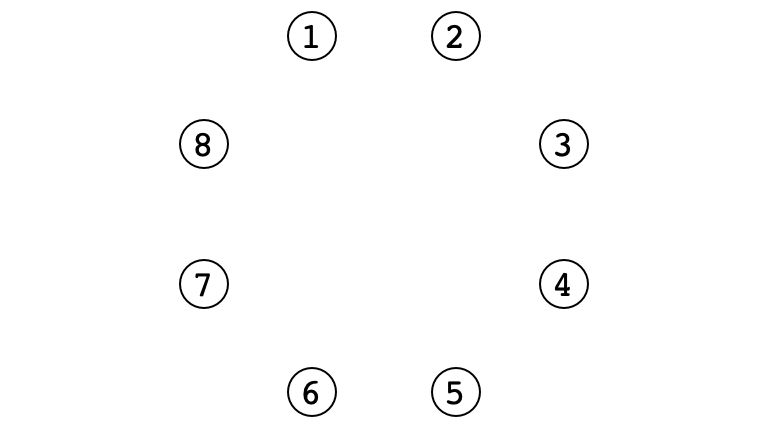A Wrinkle

Josephus does not want a manicure!

Question. Where should Josephus sit at the table to ensure that he does not receive a manicure?

An Activity

• Devise a procedure for determining where Josephus should sit to avoid a manicure

• Use a queue!

• assume your queue has a getSize() method